# Eliminating a Bessel function branch cut

In an earlier post I looked in detail at a series for inverse cosine centered at 1. The function arccos(z) is multivalued in a neighborhood of 1, but the function

arccos(z) / √(2 – 2z)

is analytic in a neighborhood of 1. We cancel out the bad behavior of inverse cosine at 1 by dividing by another function with matching bad behavior.

We can do something similar with Bessel functions. In general, the Bessel function Jν has a branch cut from -∞ to 0. But the function

Jν / zν

is entire, i.e. analytic everywhere in the complex plane. From a certain perspective—namely the perspective advocated by Bille Carlson (1924–2013)—we got the definition of Bessel functions (and other functions) wrong. Carlson’s book Special Functions of Applied Mathematics shows how many special functions can be defined in terms of a smaller number of functions with fewer branch cuts. This simplification revealed symmetries that hadn’t been noticed before.

Here’s a plot of J1/3(z). Height represents magnitude and color represents phase.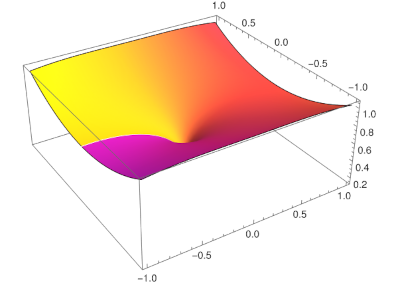This was produced using the Mathematica command

    ComplexPlot3D[BesselJ[1/3, z], {z, -1 - I, 1 + I}]

The abrupt change from purple to yellow along the negative real axis shows the discontinuity in the phase at the branch cut. You can also see a hint of the cusp at the origin.

Here’s a plot of J1/3(z) / z1/3.ComplexPlot3D[BesselJ[1/3, z]/z^(1/3), {z, -1 - I, 1 + I}]

The white streak is an artifact of the branch cuts for J1/3(z) and for z1/3. The branch cut in their ratio is unnecessary.

# Complex AGM

The arithmetic-geometric mean (AGM) of two non-negative real numbers a and b is defined as the limit of the iteration starting with a0 = a and b0 = b and

an+1 = ½ (an + bn)
bn+1 = √(an bn)

for n > 0. This sequence converges very quickly and is useful in numerical algorithms. The limit can be expressed in terms of an elliptic function, and that elliptic function can then be related to other functions. See, for example, this post for how the AGM can be used to compute logarithms to arbitrary precision.

Since the AGM is useful in computing special functions, and we’re often interested in evaluating special functions at complex values, it’s natural to want to evaluate the AGM for complex numbers.

But we immediately run into a difficulty: which square root do we pick to find the new b?

For a non-negative real number x, √x is defined to be the non-negative real number y such that y² = x. But for more general values of x we have to choose a branch of the square root function. Which branch should we use in the AGM?

Often when we need to take the square root of complex numbers we can use the “principal branch,” the branch that gives positive values for positive real inputs and extends to the rest of the complex plane with the negative axis removed. If you compute the square root of a complex number in software, as we’ll do below, this is probably the value you’ll get by default.

But it turns out we cannot simply pick the principal branch. Gauss discovered two centuries ago that the right branch to take could vary at each step . What does that even mean? How do we know we’ve made the “right” choice?

For one thing, we want our iteration to converge. And for another, we’d like it to converge to something non-zero if we start with non-zero inputs . The right choice will guarantee this .

So what is this right choice? We provisionally update a and b as above, using either square root for b, and keep the value of b if

|ab| ≤ |a + b|

or

|ab| = |a + b|

and the imaginary part of b/a is positive. In other words, chose the possible value of b that’s closer to a, and use the imaginary part of b/a as a tie breaker.

Here is the AGM implemented in Python, using the right square root at each step.

    def right(a, b):
d = abs(a + b) - abs(a - b)
return d > 0 or d == 0 and (b/a).imag > 0

def agm(a, b):
while abs(a-b) > 1e-14:
a1 = 0.5*(a+b)
b1 = np.sqrt(a*b)
if not right(a1, b1):
b1 = -b1
a, b = a1, b1
return a1


The test d == 0 should make you concerned. Testing floating point numbers for exact equality with zero is seldom the right thing to do, but we’ll gloss over numerical issues for this post.

Here’s an example, giving the a values of the iteration starting with 7 + 30i and 20 + 22i. The iteration converges in four steps.

    13.500000000000000 + 26.000000000000000j
13.784944719026262 + 26.397404494892115j
13.783557503026870 + 26.395953326186888j
13.783557473769877 + 26.395953309190112j


In this example, the right root is the principal root every time. To find an example where the other root is chosen, I generated random starting points until I found one that took an alternate root.

Here are the values of b starting with -1.654 – 1.178i and 2.244 – 1.956i. An asterisk indicates that the principal root was not the right root.

     0.2328790598285062 - 0.728412421988127j
-0.6829999999999998 - 0.589000000000000j
-0.2254063569280081 - 0.799311791579126j *
-0.2250604700857468 - 0.658706210994063j
-0.2261796153095098 - 0.725905503054624j *
-0.2252334135068774 - 0.729009001286595j
-0.2257078598391289 - 0.727456168426402j *
-0.2257065144081936 - 0.727457252170610j
-0.2257071871240875 - 0.727456710298747j *
-0.2257071871236612 - 0.727456710298506j
-0.2257071871238743 - 0.727456710298626j *
-0.2257071871238744 - 0.727456710298626j


## More AGM posts

 David Cox. The Arithmetic-Geometric Mean of Gauss. L’Énseignement Mathématique, 30 (1984), p. 275–330.

 If a = –b we get zero immediately. But if a and b are both not zero, and a does not equal –b, then taking the right square root at each iteration gives us what we want.

 Interestingly, you could make a finite number of wrong choices and still end up with something that might converge, albeit to a different value. This gives you a different branch of the AGM.

# Keyhole contour integrals

## The big idea

The Cauchy integral theorem says that the integral of a function around a closed path in the complex plane depends only on the poles of the integrand inside the path. You can change the path itself however you like as long as you don’t change which poles are inside. This observation is often used to compute real integrals using complex analysis.

Suppose you want to integrate a function f along (some portion of) the real line, and f extends to a function in the complex plane that is analytic except at poles. You may be able to evaluate your real integral using a complex contour, or more commonly, a limit of contours. You show that in some limit, the contribution to the integral along the parts of the contour you don’t need goes to zero, and the rest of the contour approaches the part you wanted to integrate over initially.

## Keyhole contours

There area a handful of integration contours that come up most frequently, and the keyhole is one of them. The initial motivation for this post was the cover of the fourth edition of A Course of Modern Analysis by Whittaker and Watson. I’ve written a couple posts lately about the story behind images on book covers, and this is another post in that series.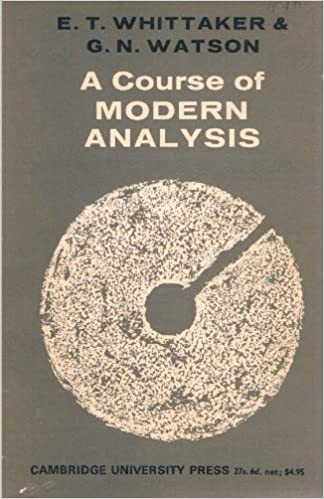The most recent edition has a more prosaic cover with only words and no image. However, the latest addition is much more attractive inside, having been rewritten in LaTeX.

The image on the cover is a keyhole contour. Typically the slot in the contour runs along the real axis, but the cover rotated the image 45 degrees to make it more visually appealing. The contour is used on page 118 of the fourth edition to integrate rational functions along the positive real axis .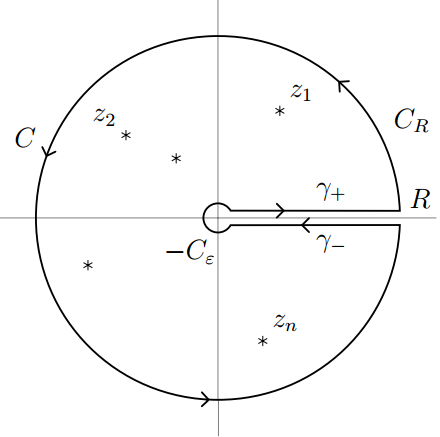If the function you’re integrating has poles only at the locations marked by stars, then you can evaluate the integral around the contour by computing the residues of the integrand at these points. Now suppose you change the contour by making the outer circle larger, letting its radius R go to infinity, and making the inner circle shrink, letting its radius ε go to zero. If the integral along these two circular segments goes to zero in the limit, you’re left with the integral along the positive real axis.

## Hankel

The keyhole contour is sometimes called the Hankel contour because Hermann Hankel used it in the 19th century to investigate special functions such as the gamma function and the eponymous Hankel functions. The first post in this series of book cover commentaries mentioned a book that has a plot of a Hankel function on the cover.

## Related posts

 Specifically, Whittaker and Watson show how to compute the integral of xa-1 Q(x) from 0 to infinity, where Q is a rational function with no positive real zeros, and the limit of xa Q(x) is zero both as x goes to zero and as x goes to ∞.

# NASA and conformal maps

A couple years ago I wrote about how NASA was interested in regions bounded by curves of the formFor example, here’s a plot for A = 2, B = 1, α = 2.5 and β = 6.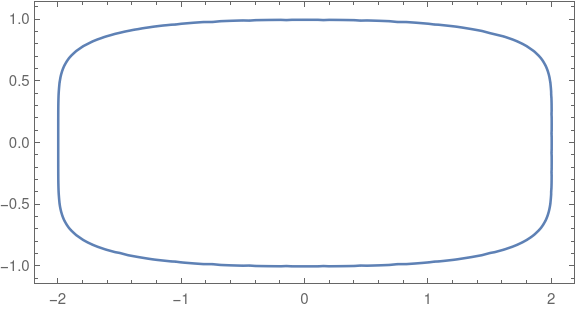That post mentioned a technical report from NASA that explains why these shapes are important in application, say for beams and bulkheads, and gives an algorithm for finding conformal maps of the unit disk to these shapes. These shapes are also used in graphic design, such as squircle buttons on iPhones.

Note that these shapes are not rounded rectangles in the sense of a rectangles modified only at the corners. No segment of the sides is perfectly flat. The curvature smoothly decreases as you move away from the corners rather than abruptly jumping to 0 as it does in a rectangle rounded only at the corners. Maybe you could call these shapes continuously rounded rectangles.

## Conformal maps

Conformal maps are important because they can map simple regions to more complicated regions in way that locally preserves angles. NASA might want to solve a partial differential equation on a shape such as the one above, say to calculate the stress in a cross section of a beam, and use conformal mapping to transfer the equation to a disk where the calculations are easier.

## Coefficients

The NASA report includes a Fortran program that computes the coefficients for a power series representation of the conformal map. All the even coefficients are zero by reasons of symmetry.

Coefficients are reported to four decimal places. The closer the image is to a circle, i.e. the closer α and β are to 2 and the closer A is to B, the fewer non-zero coefficients the transformation has. You could think of the number of non-zero coefficients as a measure how hard the transformation has to work to transform the disk into the desired region.

There are interesting patterns in the coefficients that I would not have noticed if I had not had to type the coefficients into a script for the example below. Maybe some day I’ll look more into that.

## Conformal map example

The following example uses A = 2, B = 1, and α = β = 5.And here is the image of the disk under the conformal map giving by the coefficients in the NASA report.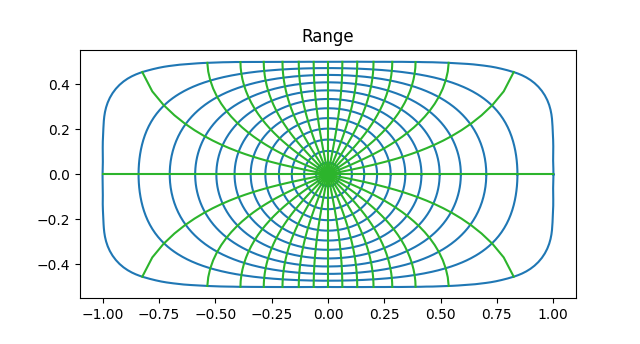Note that the inner most circles from the domain are mapped into near circles, but as you move outward the circles are distorted more, approaching the shape of the boundary of the range.This is because in the limit, analytic functions map disks to disks. So a tiny circle around the origin will always map to an approximate circle, regardless the target region.

Note also that although the circles and lines of the domain are warped in the range, they warp in such a way that they still intersect at right angles. This is the essence of a conformal map.

## Inverse map

The NASA report only gives a way to compute the transformation from the disk to the target region. Typically you need to be able to move both ways, disk to target and target to disk. However, since the transformation is given by a power series, computing the inverse transformation is a problem that has been solved in general. This post gives an explanation and an example.

# Transformations of Olympic rings

The previous post gave the details of how Möbius transformations

m(z) = (az + b)/(cz + d)

transform circles. The image of a circle under a Möbius transformation is either a line or a circle, and in our examples the image will always be a circle.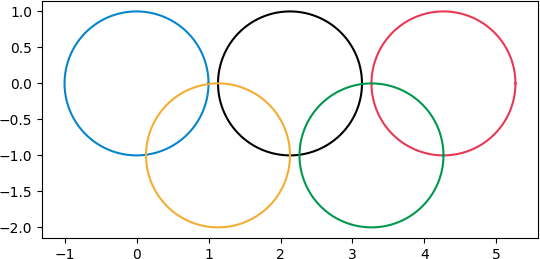and then apply various transformations.

First we apply (z + 9)/(z + 10):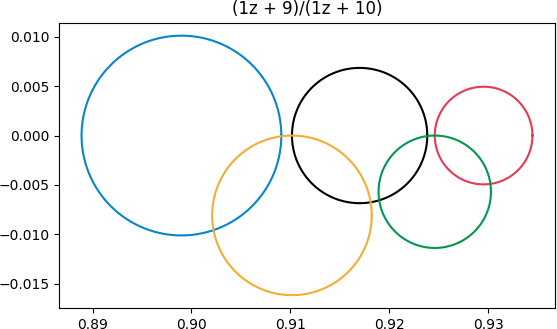This transformation doesn’t distort the rings too much because the rings are far from the singularity at z = -10. The blue ring is distorted the most because it’s closest to the singularity.

Next we apply (iz + 7)/(z + 8).  Now we’ve moved the singularity a little bit closer, and we caused some rotation by using i as the coefficient of z in the numerator.Finally, let’s pick a transformation with its singularity inside the green circle, (z + 4)/(z -3 + i).Now we see a lot of distortion. The blue and gold rings that were outside the green ring are brought inside. The complex plane is turned inside-out because of the singularity.

As before, the black and red rings still intersect the green ring. But what you can’t tell from the graph is that the portion of these rings that was inside has been turned outside and vice versa.

# Circles and lines under a Möbius transformation

This post will revisit a previous post in more detail. I’ve written before about how Möbius transformations map circles and lines to circles and lines. In this post I’d like to explore how you’d calculate which circle or line a given circle or line goes to. Given an equation of a line or circle, what is the equation of its image?

## Circles to circles

A while back I wrote a post called Circles to Circles, demonstrating how Möbius transformations map circles and lines to circles and lines. If you think of a line as a degenerate circle, a circle of infinite radius, then you could simply say Möbius transformations map circles to circles.

For example I showed in that post that the transformation

m(z) = (z + 2)/(3z + 4)

maps these circlesto these circles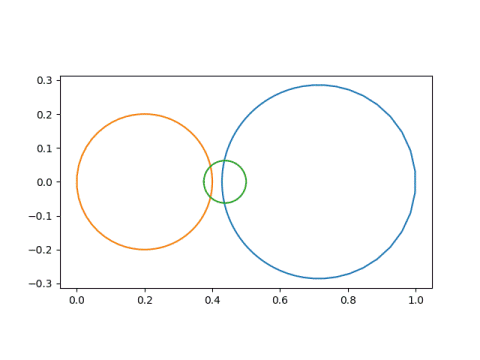and that these lines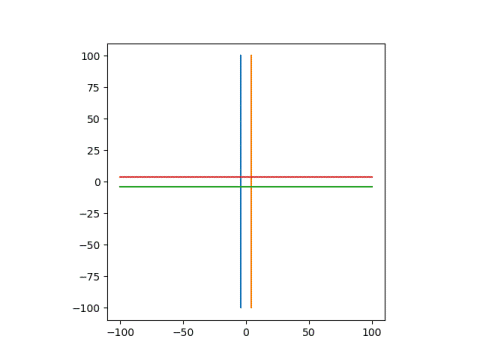go to these circles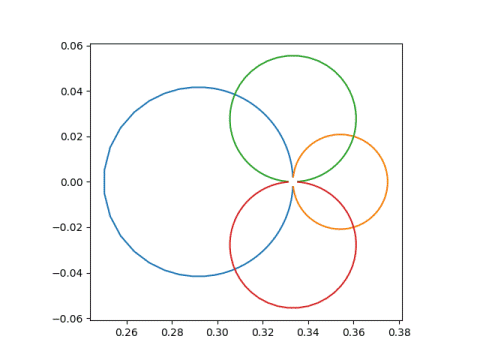## Circle or line image

Given the equation of a circle or a line, how would you know whether its image is a circle or a line?

A Möbius transformation

m(z) = (az + b) / (cz + d)

has a singularity at z = –d/c. If a line or curve goes through the point –d/c then its image is a line. Otherwise the image is a circle.

If the line or curve does go through –d/c, so that the image is a line, it’s easy to find an equation of the line. Pick two points, z1 and z2 on the original curve or line. Let w1m(z1) and w2m(z2). Then find the equation of a line through w1 and w2. For example, a parametric form is

w1 + t(w2w1)

for all real t.

## Equation of the image circle

m(z) = (az + b) / (cz + d)

as above, but we assume our circle does not pass through –d/c since that case is covered above. With this assumption we can conclude that the image of our circle is another circle, and the remaining task is to find an equation of that circle.

We can decompose our transformation m into several simpler steps—translation, reciprocal, rotation, and dilation—and see what happens to the center and radius under each step. The hardest step is taking the reciprocal, and this is covered in the previous post. That post was a lemma for this post.

We can apply m to z in four steps:

2. Take the reciprocal.
3. Multiply by e = (bcad)/c².

We know it’s OK to take the reciprocal in the second step because our circle does not contain the point z = –d/c.

We can break step 3 into (3a) multiplying by |e| and (3b) multiplying by e/|e|. Step 3a is a dilation and step 3b is a rotation.

Here’s a Python program using the steps above to find the center and radius of a transformed circle.

    def transform(a, b, c, d, center, radius):
# step 1
center += d/c

# step 2
temp = center
center = np.conj(center) / (abs(center)**2 - radius**2)

# step 3
e = (b*c - a*d)/c**2
center *= e

# step 4
center += a/c



# Computing zeta at even numbers

Last year I wrote several posts about computing ζ(3) where ζ is the Riemann zeta function. For example, this post.

It happens that ζ can be evaluated in closed form at positive even arguments, but there’s still a lot of mystery about zeta at positive odd arguments.

There’s a way to derive ζ(2n) using contour integration. But you don’t need to be clever about constructing contours if you start from the following result which is derived using contour integration.

Suppose f is analytic except for poles at zk and that the sumconverges, where the sum is over all integer n except poles of f. Then the sum can be computed from residues at the poles:Here Res stands for “residue.” The residue of a function g(z) at z0 is defined as the coefficient c-1 of (zz0)-1 in the Laurent series for g. Equivalently,This means we can evaluate infinite sums by taking a limit that may be a simple application of L’Hôpital’s rule.

The theorem above could be used to calculate a lot more than values of the Riemann zeta function by choosing various functions f, but for our purposes f(z) will be z-2m for some positive integer m.Why can’t this same trick be used for evaluating the Riemann zeta function at odd integer arguments? The second equality above fails. For even powers of n, the sum over the positive integers is half the sum over all integers. For odd powers, the sum over all integers is zero.

If we write out the Laurent series for π cot πz then we can read off the residues of π cot πz/z2m.When we divide by z² the coefficient of z becomes the coefficient of z-1, i.e. the residue. This residue is -π²/3, and so negative one half of this is π²/6, i.e. ζ(2) = π²/6.

The same line of reasoning shows that ζ(4) = π4/90 and ζ(6) = π6/945.

Finding the values of ζ(2m) in general is no harder than finding the power series for cotangent, which is not trivial but not insurmountable either. See this post.

The final result is that# Constructive Picard

The previous post concerned the function

h(z) = exp(-1/(1 – z² )).

We said that the function is badly behaved near -1 and 1. How badly?

The function has essential singularities at -1 and 1. This means that not only does h blow up near these points, it blows up spectacularly.

Picard’s theorem says that if a meromorphic function f has an essential singularity at z0, then near z0 the function takes on every value in the complex plane infinitely often. That’s almost true: Picard’s theorem says f can miss one point.

Suppose we take a little disk around 1 and a complex number w. Then Picard’s theorem says that there are infinitely many solutions to the equation

h(z) = w

inside the disk, except possibly for one value of w. Since the exponential function is never 0, we know that 0 is the one value h cannot take on. So for any non-zero w, we can find an infinite number of solutions to the equation above.

OK, so let’s actually do it. Let’s pick a w and look for solutions. Today’s June 24, so let’s pick w = 6 + 24i. We want to find solutions to

exp(-1/(1 – z²)) = 6 + 24i.

Taking the log of both sides, we need to find values of z such that

-1/(1 – z²) = log(6 + 24i)

This step should make you anxious. You can’t just take logarithms of complex numbers willy nilly. Which value of the logarithm do you mean? If we pick the wrong logarithm values, will we still get solutions to our equation?

Let’s back up and say what we mean by a logarithm of a complex number. For a non-zero complex number z, a logarithm of z is a number w such that exp(w) = z. Now if z is real, there is one real solution w, hence we can say the logarithm in the context of real analysis. But since

exp(w + 2πin) = exp(w)

for any integer n, we can add a multiple of 2πi to any complex logarithm value to get another.

Now let’s go back and pick a particular logarithm of 6 + 24i. We’ll use the “principal branch” of the logarithm, the single-valued function that extends the real logarithm with a branch cut along the negative real axis. This branch is often denoted Log with a capital L. We have

Log(6 + 24i) = 3.20837 + 1.32582i.

When we take the logarithm of both sides of the equation

exp(-1/(1 – z²)) = 6 + 24i.

we get an infinite sequence of values on both sides:

in – 1/(1 – z²) = 2πim + Log(6 + 24i)

for integers n and m. For each fixed value of n and m the equation above is a quadratic equation in z and so we can solve it for z.

Just to make an arbitrary choice, set n = 20 and m = 22. We can then ask Mathematica to take solve for z.

    NSolve[40 Pi I - 1/(1 - z^2) == 44 Pi I Log[6 + 24 I], z]

This gives two solutions:

z = -0.99932 + 0.00118143i

and

z = 0.99932 – 0.00118143i.

In hindsight, of course one root is the negative of the other, because h is an even function.

Now we don’t need n and m in

in – 1/(1 – z²) = 2πim + Log(6 + 24i)

because we can consolidate the move 2πim to the left side and call nm the new n. Or to put it another way, we might as well let m = 0.

So our solutions all have the form

in – Log(6 + 24i) = 1/(1 – z²)

z² = 1 + 1/(Log(6 + 24i) – 2πin).

The larger n gets, the closer z gets to 1. So this shows constructive what Picard said would happen: we have a sequence of solutions converging to 1, so no matter how small a neighborhood we take around 1, we have infinitely many solutions in that neighborhood, i.e. h(z) = 6 + 24i infinitely often.

# No analytic bump

The word “smooth” in mathematics usually means infinitely differentiable. Occasionally the word is used to mean a function has as many derivatives as necessary, but without being specific about how many derivatives that is.

A function is analytic if it has a convergent power series representation at every point of its domain. An analytic function is infinitely differentiable, but surprisingly the converse is not true.

Suppose we took a bump function as described in the previous post, say over the interval [-1, 1]. Such a function would be infinitely differentiable at 1, positive to the left of 1 and zero to the right. Let’s compute the power series centered at 1. All the derivatives are zero at 1, so all the power series coefficients are 0, so the power series sums to 0. The bump function is positive on the left side of 1 but the power series is not.

When does the power series of an infinitely differentiable function converge to that function? More on this subtle question here.

Now suppose we forge ahead and try to construct an analytic bump function. What goes wrong? Do we get something that is almost analytic?

Let’s follow the construction of the previous post, starting with

f(x) = exp(x).

Then

g(x) = 1 /f(1/x) = exp(-1/x)

and

h(x) = g(1+x) g(1-x) = exp(1/(1 – x²))

In Mathematica,

    h[x_] := If[Abs[x] < 1, Exp[-1/(1 - x^2)], 0]

Now let’s look at h from several perspectives. Along the real axis, everything is fine.

    Plot[h[x], {x, -1.1, 1.1}]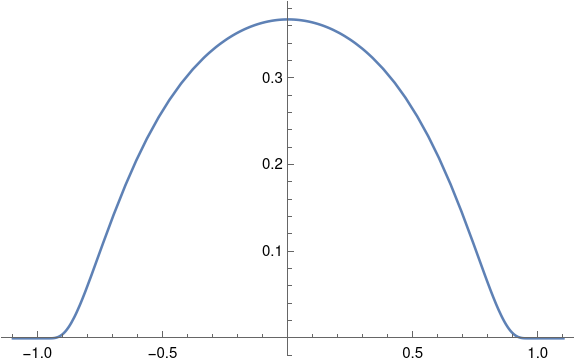The function seems to drop to zero abruptly so that it might not be smooth, but zooming in a little reassures us that the function is indeed smooth.

    Plot[h[x], {x, -1.1, -0.9}, PlotRange -> All]What if we plot h as a complex function?Now things don’t look so smooth. The swirls of color near -1 and 1 show that the phase is changing violently. Also, the light color in the unit circle compared to the dark color outside suggests the phase jumps when we cross the unit circle rather than smoothly transitioning to zero.

Let’s see what happens to the magnitude of h as we cut across the square above diagonally.

    Plot[Abs[h[z I + z]], {z, -1, 1}]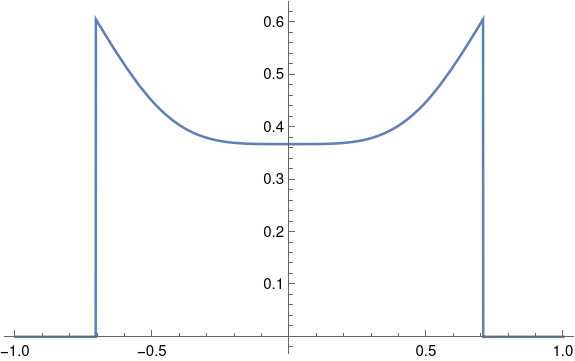Definitely not smooth. Not even continuous.

Let’s see what happens if we add a dimension to our plot, using |h(z)| as height.

    ComplexPlot3D[h[z], {z, -1.1 - 1.1 I, 1.1 + 1.1 I}]So a lot is happening at ±1. Let’s turn this plot around to look at it from a different perspective.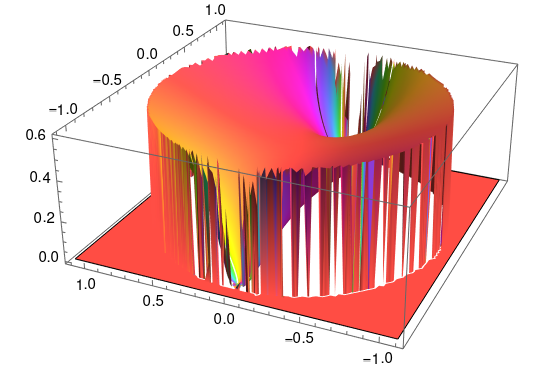Our function has essential singularities at ±1 when we look at it in the complex plane. Along the real axis everything looks fine, but when we look at it from the complex plane we see that the function is very badly behaved.

Before we go, let’s go back and look at the example from the previous post, a bump function that is six derivatives along the real axis. What would it look like extended to the complex plane?

    h2[z_] := If[Abs[z] < 1, (1 + z)^7 (1 - z)^7, 0]
ComplexPlot3D[h2[z], {z, -1.1 - 1.1 I, 1.1 + 1.1 I}]


This gives us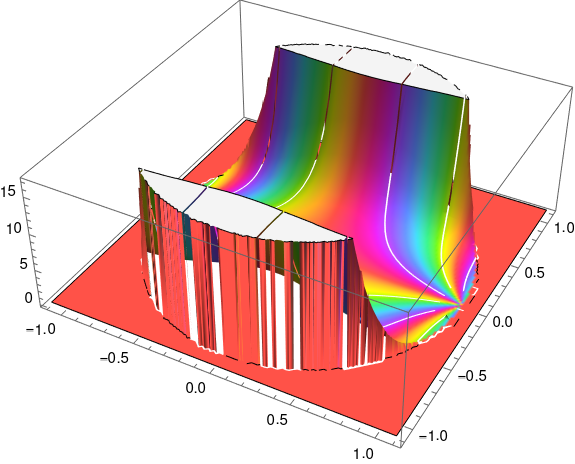Here's the same plot viewed from a different angle.# Doubly periodic but not analytic

A sine wave is the canonical periodic function, so an obvious way to create a periodic function of two variables would be to multiply two sine waves:

f(x, y) = sin(x) sin(y)

This function is doubly periodic: periodic in the horizontal and vertical directions.

Now suppose you want to construct a doubly periodic function of a complex variable z = x + iy. One thing you might try is

f(x + iy) = sin(x) sin(y)

This is a function of a complex variable, technically, but it’s not what we usually have in mind when we speak of functions of a complex variable. We’re usually interested in complex functions that are differentiable as complex functions.

If h is the increment in the definition of a derivative, we require the limit as h approaches 0 to be equal no matter what route h takes. On the real line, h could go to zero from the left or from the right. That’s all the possibilities. But in the complex plane, h could approach the origin from any angle, or it could take a more complex route such as spiraling toward 0.

It turns out that it is sufficient that the limit be the same whether h goes to 0 along the real or imaginary axis. This gives us the Cauchy-Riemann equations. If

f(x, y) = u(x, y) + i v(x, y)

then the Cauchy-Riemann equations require the partial derivative of u with respect to x to equal the partial derivative of v with respect to y, and the partial derivative of v with respect to x to be the negative of the partial of u with respect to y.

ux = vy
vx = –uy

These equations imply that if v = 0 then u is constant. A real-valued function of a complex variable cannot be analytic unless its constant.

So can we rescue our example by making up an imaginary component v? If so

f(x, y) = sin(x) sin(y) + i v(x, y)

would have to satisfy the Cauchy-Riemann equations. The first equation would require

v(x, y) = – cos(x) cos(y) + a cos(x)

for some constant a, but the second would require

v(x, y) =  cos(x) cos(y) + cos(y)

for some constant b. Note the negative sign in the former but not in the latter. I’ll leave it as an exercise for the reader to show that these requirements are contradictory.

Liouville’s theorem says that a bounded analytic function must be constant. If an analytic function f is doubly periodic, then it must either be constant or have a singularity. There are many such functions, known as elliptic functions.